## A rectangular swimming pool is twice as long as it is wide. A small concrete walkway surrounds the pool. The walkway is a 2 feet wide and ha

Question

A rectangular swimming pool is twice as long as it is wide. A small concrete walkway surrounds the pool. The walkway is a 2 feet wide and has an area of 448 square feet including the pool.Find the dimensions of the pool

in progress 0
2 weeks 2021-08-30T19:01:39+00:00 1 Answers 0 views 0

The width and the length of the pool are 12 ft and 24 ft respectively.

Step-by-step explanation:

The length (L) of the rectangular swimming pool is twice its wide (W):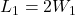Also, the area of the walkway of 2 feet wide is 448: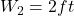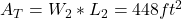Where 1 is for the swimming pool (lower rectangle) and 2 is for the walkway more the pool (bigger rectangle).

The total area is related to the pool area and the walkway area as follows: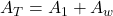(1)

The area of the pool is given by: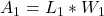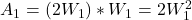(2)

And the area of the walkway is: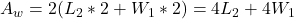(3)

Where the length of the bigger rectangle is related to the lower rectangle as follows: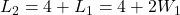(4)

By entering equations (4), (3), and (2) into equation (1) we have: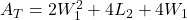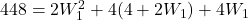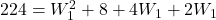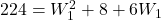By solving the above quadratic equation we have:

W₁ = 12 ft

Hence, the width of the pool is 12 feet, and the length is: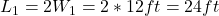Therefore, the width and the length of the pool are 12 ft and 24 ft respectively.

I hope it helps you!